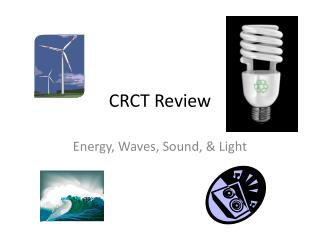DownloadDownload PresentationCRCT Review

# CRCT Review

Download Presentation## CRCT Review

- - - - - - - - - - - - - - - - - - - - - - - - - - - E N D - - - - - - - - - - - - - - - - - - - - - - - - - - -
##### Presentation Transcript

1. CRCT Review Energy, Waves, Sound, & Light

2. Convection currents do NOT take place in _________________. • A) liquids • B) gases • C) solids • D) the ocean

3. The transfer of heat by collision of atoms in a solid is… • A) conduction • B) convection • C) radiation • D) Thermal energy

4. You and your friends are telling ghost stories around a campfire. What type of heat transfer are you using? • A) convection • B) conduction • C) radiation • D) insulation

5. In a vacuum (open space), heat transfer occurs by • A) Conduction • B) Convection • C) Radiation • D) Thermal Energy

6. On Earth, cool air circulates to warm regions and warm air circulates to cooler regions. What type of heat transfer does this describe? • A) conduction • B) Convection • C) Radiation • D) Fahrenheit

7. Electricity is a type of ______. • A) energy • B) machine • C) matter • D) light

8. Waves transfer _____. • A) matter • B) energy • C) particles • D) water

9. Long wavelength makes _____ frequency. • A) high • B) low • C) zero • D) fast

10. According to Doppler Dan, if a sound is moving toward you then the pitch is ___________. • A) Low • B) High • C) loud • D) soft

11. According to Doppler Dan, if a sound is moving away from you then the pitch is ___________. • A) low • B) high • C) loud • D) soft

12. The wave property that is related to the height of a wave is the _____. • A) wavelength • B) amplitude • C) frequency • D) wave speed

13. Waves that don’t require a medium are __________. • A) longitudinal waves • B) electromagnetic waves • C) surface waves • D) mechanical waves

14. When you look in the mirror, what type of light behavior are you observing? • A) refraction • B) reflection • C) diffraction • D) interference

15. How does a prism make a rainbow? • A) refraction • B) reflection • C) diffraction • D) interference

16. You walk down a dark hallway and suddenly a light spills out of an open doorway. What light wave behavior have you experienced? • A) Reflection • B) Refraction • C) Interference • D) Diffraction

17. When light is refracted, the visible color spectrum is displayed. What is the acronym we use to remember the colors? • A) PEMDAS • B) ROYGBIV • C) MVEMJSUN • D) SONAR

18. Light waves are more than just the light we see. What is another kind of light wave that we DON’T see? • A) red • B) sun • C) radio • D) blue

19. Hand Lens Problem Solving • Teachers sometimes use overhead projectors to show transparencies on a screen. What type of lens does an overhead projector use? Convex Concave Flat-plane eye

20. How do electromagnetic waves emitted by the sun heat the Earth? • A) conduction • B) convection • C) radiation • D) insulation

21. What type of wave interaction is an echo? • A) reflection • B) refraction • C) diffraction • D) interference

22. What are the three types of mirrors and lenses? • A) cone, rectangle, oval • B) curved, flat, round • C) concave, convex, plane • D) square, concave, convex

23. If you are farsighted, you cannot see _____ and you need a ____ lens. • A) far away, concave • B) far away, convex • C) close-up, concave • D) close-up, convex

24. If you are nearsighted, you cannot see _____ and you need a ____ lens. • A) far away, convex • B) far away, concave • C) far away, convex • D) far away, concave